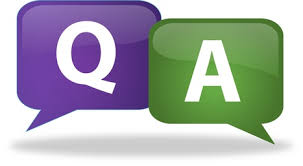# SCC Education

## Electricity test paperFORMATIVE ASSESSMENT I
MARKS-30
Instructions:
 Questions : 1 to 5 – 1 Mark each
 Questions : 6 to 9 – 2 Marks each
 Questions : 10 to 13 – 3 Marks each
 Question 14 – 5 Marks
1. Name the instrument used to measure electric current.
2. What is the mathematical form of Ohm‘s law?
3. Name a metal which is the best conductor electricity.
4. Why resistance become more in series combination?
5. What is electrical power? Write its unit.
6. Why does resistance of a conductor increase with increase in temperature?
7. Current in a circuit is doubles by changing resistance. What will be the change in power loss?
8. Why are the conductors of electric heating devices such as bread toaster and electric iron made of alloy rather than pure metal?
9. Draw the following symbols
i) Electric cell ii) Switch open iii) Electric bulb iv)Ammeter
10. A 100 watt electric bulb is lighted for 2 hours daily and four 40 watt bulbs are lighted for 4 hours daily. Calculate the energy consumed (in kWh) in 30 days.
11. Resistance of a conductor depends on which three factors. Explain with mathematical expression.
12. What is the effect of temperature on resistance of – pure metals, insulators and semi – conductors.
13. Write 3 points of difference between direct and alternate current.
14. a) Draw diagram showing three resistors R1, R2 and R3 in parallel.
b) Two identical resistors, each of resistance 2 ohms, are connected in turn
i) In series and
ii) In parallel
To a battery of 12 volts. Calculate the ratio of power consumed in two cases.

light-reflection-refraction-objectives
The-human-eye-and-colourful-world
Human-eye-and-colourful-world
Comparison-between-ac-and-dc
Electricity-test
Magnetic-effects-of-electric
Elecricity-notes

Magnetic-effects-of-electric-current